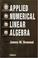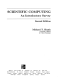### Computational Mathematics

#### Course Description

Errors in numerical computations. Numerical solution of linear systems. Interpolation and approximation. Numerical integration. Finite differences. Solving nonlinear equations. Numerical solution of ordinary differential equations.

#### General Competencies

Introduction to the basic numerical methods for solving standard numerical problems (liner systems solving, interpolation and approximation, numerical integration, numerical solutions of the systems of nonlinear equations and ordinary differential equations). Applying these methods on the real life problems, understanding and correct interpretation of obtained results.

#### Learning Outcomes

1. Recognize the type of errors which are present during the numerical solving of various real-life problems.
2. Name basic direct methods for solving linear systems. Furthermore, student will be able to chose the appropriate method and solve a given linear system
3. Identify an interpolation polinom and a spline function for given data and can estimate the approximation error
4. Calculate the approximation of the given integral and estimate the approximation error
5. Apply the fast Fourier transform on the filtration signals problems
6. Apply QR factorization in solving problems using the least square method
7. Solve a nonlinear systems using Newton method in more dimensions
8. Use basic methods of unconditioned optimization.

#### Forms of Teaching

Lectures

Lectures are held in two cycles, three hours per week

Exams

midterm and final exam, online tests

Laboratory Work

students solve exercises in Matlab or using some other software package for numerical simulations

Consultations

one hour per week

Seminars

advanced students present some themes from numerical analysis

Programming Exercises

programming exercises in Matlab, Python, Octave, Scilab or any chosen software package for numerical computing

E-learning

use the system for E-learning Moodle

#### Grading Method

Continuous Assessment Exam
Type Threshold Percent of Grade Threshold Percent of Grade
Homeworks 0 % 5 % 0 % 5 %
Quizzes 0 % 15 % 0 % 15 %
Mid Term Exam: Written 0 % 40 % 0 %
Final Exam: Written 0 % 40 %
Exam: Written 0 % 80 %

#### Week by Week Schedule

1. Errors in numerical computations. Machine representation of numbers and floating point arithmetic.
2. Introduction to linear systems solving. Method of Gaussian elimination. LU factorization.
3. Method of Gaussian elimination with partial pivoting. PLU factorization.
4. Symmetric positive definite matrices. Cholesky factorization.
5. Solution of tridiagonal systems. Error analysis for linear systems.
6. Approximation and interpolation of functions. Lagrange interpolating polynomial. Error estimation.
7. Numerical integration. Newton-Cotes formulas. Adaptive integration methods.
8. Midterm exam.
9. Linear and cubic splines.
10. Trigonometric interpolation. Discrete and inverse discrete Fourier transform. FFT algorithm.
11. Least squares method. Normal equations. QR and SVD matrix factorization.
12. Solution of nonlinear equations. Simple iteration method. Newton method. Error analysis and rate of convergence.
13. Numerical solution of nonlinear systems. Quasi-Newton methods. Introduction to optimization problems.
14. Solving ordinary differential equations. Runge-Kutta methods. Solving boundary-value problems using finite differences.
15. Final exam.

#### Study Programmes

##### University graduate
Computer Engineering (profile)
Mathematics and Science (2. semester)
Computer Science (profile)
Mathematics and Science (2. semester)
Control Engineering and Automation (profile)
Mathematics and Science (2. semester)
Electrical Engineering Systems and Technologies (profile)
Mathematics and Science (2. semester)
Electrical Power Engineering (profile)
Mathematics and Science (2. semester)
Electronic and Computer Engineering (profile)
Mathematics and Science (2. semester)
Electronics (profile)
Mathematics and Science (2. semester)
Information Processing (profile)
Mathematics and Science (2. semester)
Software Engineering and Information Systems (profile)
Mathematics and Science (2. semester)
Telecommunication and Informatics (profile)
Mathematics and Science (2. semester)
Wireless Technologies (profile)
Mathematics and Science (2. semester)

#### Literature

J.P.Milišić, A.Žgaljić Keko (2013.), Uvod u numeričku matematiku za inženjere, ElementJ. Demmel (1997.), Applied Numerical Linear Algebra, SIAMM. T. Heath (2002.), Scientific Computing: An Introductory Survey, McGrawHill, New York
A. Quarteroni, R. Sacco, F. Saleri (2007.), Numerical Mathematics, Text in Applied Mathematics, Springer, Berlin, Heildeberg

#### General

ID 34553
Summer semester
4 ECTS
L0 English Level
L2 e-Learning
45 Lectures
0 Exercises
0 Laboratory exercises
0 Project laboratory

85 Excellent
75 Very Good
60 Good
50 Acceptable